Tutorials
scala

IF ELSE in Scala

In this tutorial, you'll learn about conditional IF ELSE statements in the Scala programming language.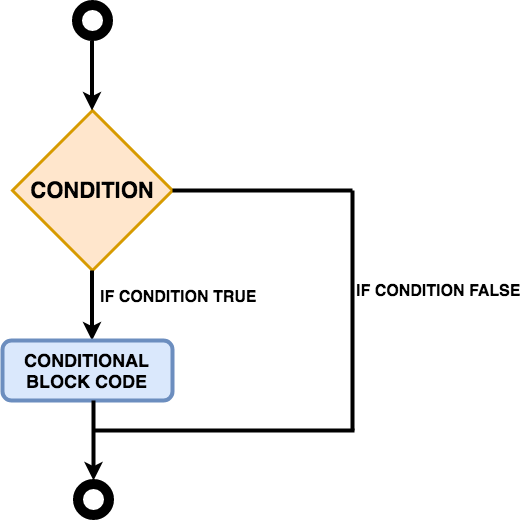Source: Scala - IF ELSE Statements

Like many other applications and programming languages, Scala also has a decision making conditional if-else statements. The if statement conditional block is executed if the condition is found to be True, if not then the else conditional block is implemented (only if, else statement is present).

Generally, the else statement has no condition; it is executed only when the if condition is False. So in short if-else statements consists of boolean expressions which work on a True-False basis.

There are various kinds of if-else statements:

- If statement,

- If-Else statement,

- Nested If-else statement,

- If-Else-If-Else statement.

Before you start learning the different if-else statements, let's quickly set up the Scala environment for mac users by following the below steps.

Note: Make sure you have Java installed, if not then please follow the instructions provided here.

- \$brew update - \$ brew install scala

- \$brew install sbt - \$ echo '-J-XX:+CMSClassUnloadingEnabled' >>
/usr/local/etc/sbtopts

- \$echo '-J-Xmx2G' >> /usr/local/etc/sbtopts Once done with the above steps quickly test the installation by typing scala in the terminal, you should see an output as shown below.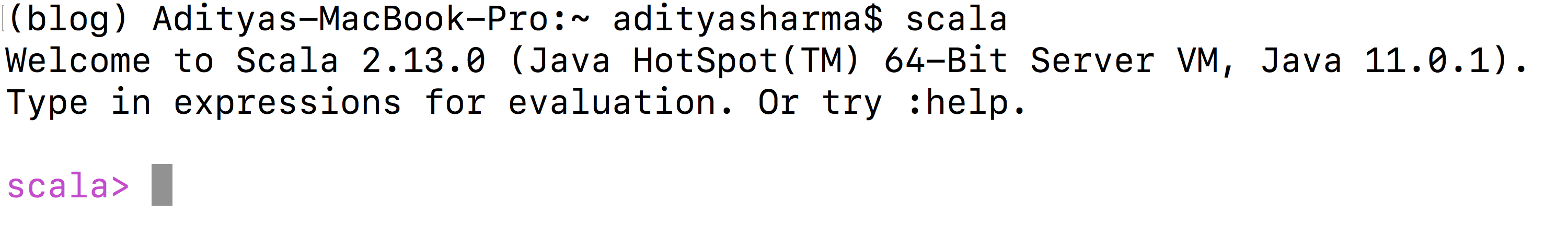Note: In this tutorial, you will be writing the code in the vim editor and executing it from the terminal. Scala if Statement The if statement comprises of an expression which is boolean (true or false) in nature. If the expression holds true, then the block of code within the if statement is executed if the expression is false, then the first line of code after the end of the if statement gets executed. if(boolean expression) { execute if boolean expression holds true } First, you will define an object or a class named scala_if inside that object you will set your main function and write your main body of the code. You will create an integer variable x with a value 20. Finally, comes the crux of the code which is the if block with a condition x<=20 and since x has a value 20, the block inside the if statement will get executed. Let's save the below code with a name if.scala.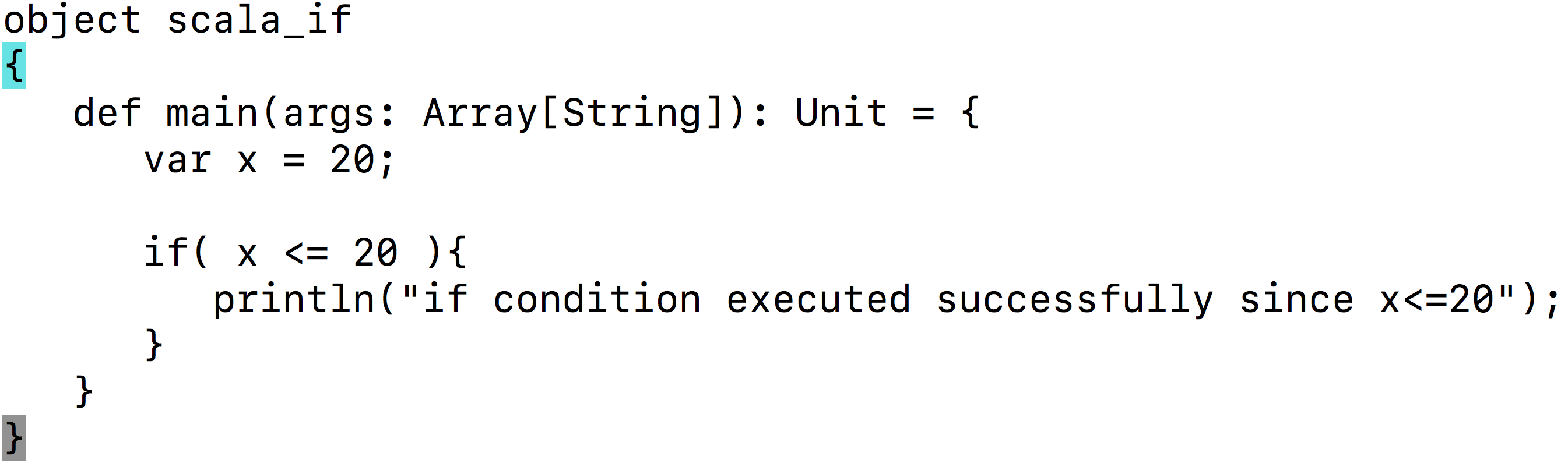To run the above code, all you need to do is type scala if.scala and you should see an output as shown below.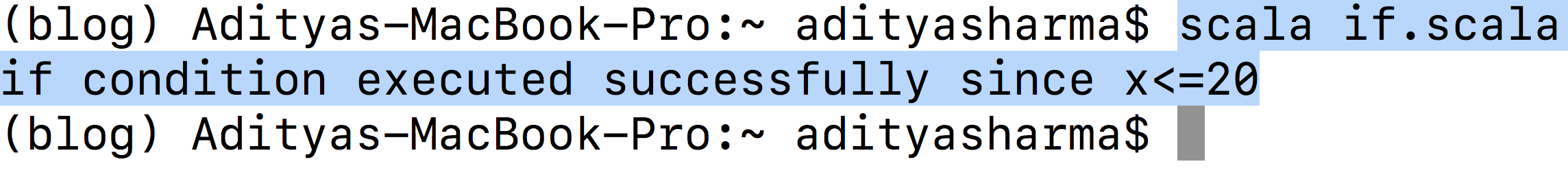Scala if-else Statement Similar to the if statement, the if-else statement comprises of an expression which is boolean (true or false) in nature. If the if statement holds true, then the block of code within the if statement is executed, if it is false then the block of code within the else statement gets executed. if(boolean expression) { execute if boolean expression holds true } else { execute block if boolean expression (if statement) holds false } The following code will execute the else statement since variable x has a value greater than 20, the block of code inside the else statement will get executed. Let's save the below code with a name of if-else.scala.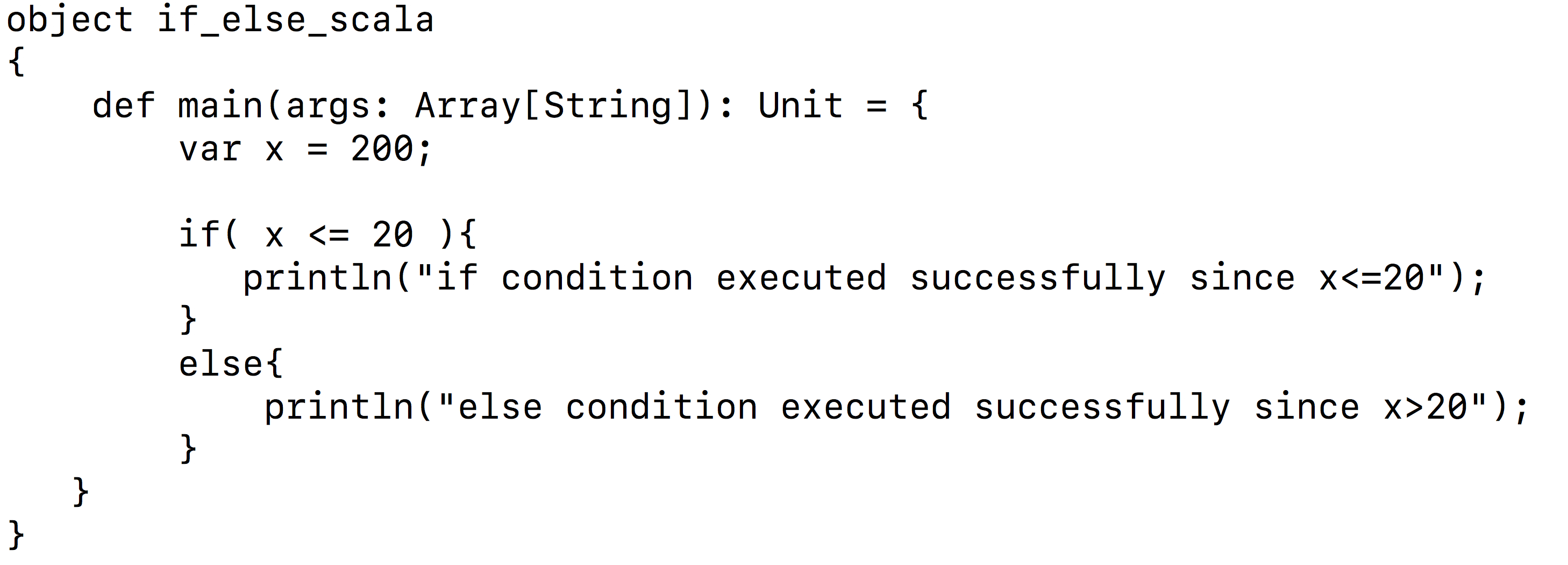To run the above code, all you need to do is type scala if-else.scala and you should see an output as shown below.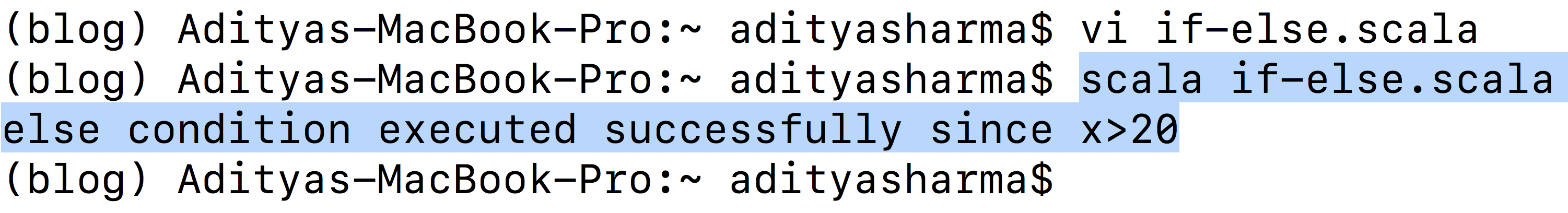Scala Nested if-else Statement Much like you usually have an if statement followed by an else statement, the nested if-else statement is also similar in spirit. In nested if-else, you can use one if or else-if statement inside another if or else-if statement. In the next section, you will be looking at the else-if statements. if(boolean expression 1) { execute block 1 if boolean expression 1 holds true** if(boolean expression 2) { execute block 2 if boolean expression 1 and 2 holds true } } The following code uses nested-if statements for comparing four integer variables namely$v$,$x$,$y$, and$z\$ and finally, if all the statements are correct, then it prints output on the terminal.

Let's save the below code with a name nested-if-else.scala.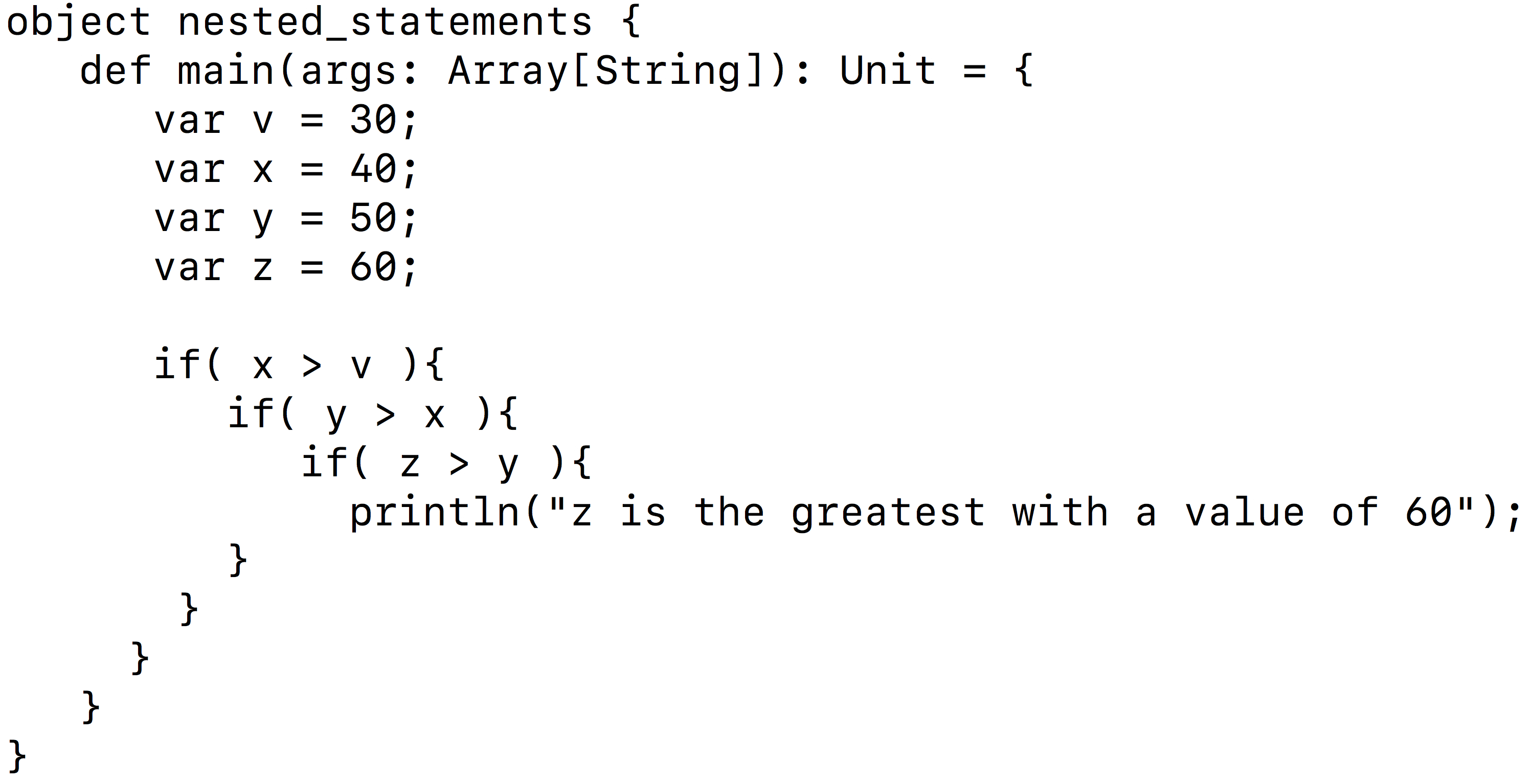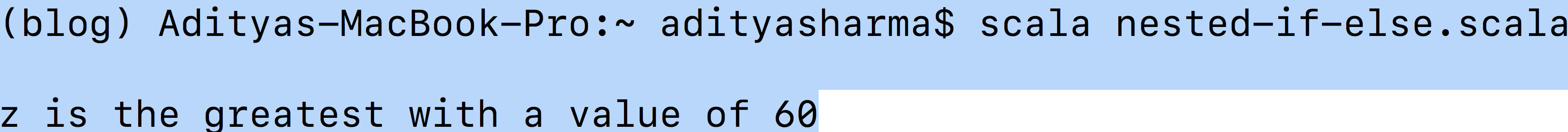Scala else-if Statement

The else-if statement is compelling compared to the if-else statements. Unlike, if-else statements wherein an else is followed by an if statement, else-if is in itself a single statement. It gives you the freedom to test various conditions.

However, there are few rules to keep in mind while using else-if statements:

• An else-if should come only after an if statement, an if statement can have zero or more else-if's.

• Similarly, an else should come only after an if and else-if statements, an if statement can have zero or one else statement.

• Once an else-if succeeds, none of the remaining else-if's or else's will be considered and will be directly skipped.

if(boolean expression 1)
{
execute block 1 if boolean expression 1 holds true
}
else-if(boolean expression 2)
{
execute block 2 if boolean expression 2 holds true
}
else
{
execute this block if all of the above statements holds false
}

Conclusion

Congratulations on finishing the tutorial.

This tutorial was a good starting point for beginners who are interested to learn the conditional IF ELSE statements in Scala.

A small exercise for you all is to write a small piece of code for the else-if statement in Scala and try to understand how these statements are different from the others.

References: